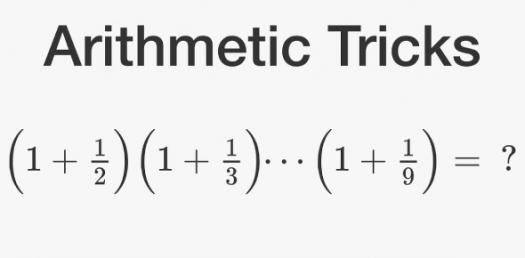# Simple Arithmetic Math Quiz Questions

Approved & Edited by ProProfs Editorial Team
At ProProfs Quizzes, our dedicated in-house team of experts takes pride in their work. With a sharp eye for detail, they meticulously review each quiz. This ensures that every quiz, taken by over 100 million users, meets our standards of accuracy, clarity, and engagement.
| Written by Alixoxo
A
Alixoxo
Community Contributor
Quizzes Created: 1 | Total Attempts: 267
Questions: 20 | Attempts: 267SettingsFor my IB maths IA. Please fill out all the questions :)

• 1.

### 2+2 =____

Explanation
The given question is a simple addition problem, asking for the sum of 2 and 2. The correct answer is 4, as when you add 2 and 2 together, the result is 4.

Rate this question:

• 2.

### 2/2=____

Explanation
The given equation 2/2 simplifies to 1. When we divide 2 by 2, the numerator and denominator are the same, resulting in a quotient of 1.

Rate this question:

• 3.

### 6-2=____

Explanation
The given equation is a simple subtraction problem where 2 is subtracted from 6. When we subtract 2 from 6, we get the result as 4. Therefore, the answer to the equation 6-2 is 4.

Rate this question:

• 4.

### 5/1=____

Explanation
The given equation 5/1=5 means that when 5 is divided by 1, the result is 5. In division, the numerator (5) is divided by the denominator (1) to give the quotient (5). Since any number divided by 1 is equal to that number itself, the answer to the equation is 5.

Rate this question:

• 5.

### 8x10=____

Explanation
The correct answer is 80 because when you multiply 8 by 10, you get the product of 80.

Rate this question:

• 6.

• 7.

### 9x5=____

Explanation
The equation 9x5=45 represents the multiplication of 9 by 5, which results in the product of 45.

Rate this question:

• 8.

### 4+4+4=____

Explanation
The equation 4+4+4 represents the addition of three numbers, each of which is 4. When we add these numbers together, we get a sum of 12.

Rate this question:

• 9.

### 7x3=____

Explanation
The equation 7x3 is asking for the product of 7 multiplied by 3. When we multiply 7 by 3, we get the result of 21. Therefore, the correct answer is 21.

Rate this question:

• 10.

### 30/6=____

Explanation
The given equation 30/6 simplifies to 5. When 30 is divided by 6, the result is 5.

Rate this question:

• 11.

### 3x4=____

Explanation
The equation 3x4=12 represents the multiplication of 3 by 4, which equals 12.

Rate this question:

• 12.

### 6-3=____

Explanation
The equation 6-3 means subtracting 3 from 6. When we subtract 3 from 6, we are left with the value of 3. Therefore, the answer to the equation 6-3 is 3.

Rate this question:

• 13.

### 1/1=____

Explanation
The equation 1/1 simplifies to 1. This is because when dividing a number by itself, the result is always 1. In this case, dividing 1 by 1 gives us 1 as the answer.

Rate this question:

• 14.

### 18/6=____

Explanation
The given equation 18/6 can be solved by dividing 18 by 6, which equals 3.

Rate this question:

• 15.

### 10+4=____

Explanation
The given equation states that when you add 10 and 4 together, the result is 14.

Rate this question:

• 16.

### 46+43=____

Explanation
The given equation is 46+43=89. When we add 46 and 43, we get a sum of 89.

Rate this question:

• 17.

### 47-18=____

Explanation
The given equation is a subtraction problem. Subtracting 18 from 47 gives a result of 29.

Rate this question:

• 18.

### 24/4=____

Explanation
The given equation is a simple division problem. When we divide 24 by 4, we get 6 as the quotient. So, the answer to the equation is 6.

Rate this question:

• 19.

### 8-6=____

Explanation
The given equation is 8-6, which means subtracting 6 from 8. When we subtract 6 from 8, we are left with 2. Therefore, the answer to the equation 8-6 is 2.

Rate this question:

• 20.

### 8x8=____

Explanation
The equation 8x8 represents the multiplication of 8 by itself. When you multiply 8 by 8, the result is 64.

Rate this question:

Related TopicsBack to top
×

Wait!
Here's an interesting quiz for you.